Disclosure: This post may contain affiliate links, meaning when you click the links and make a purchase, we receive a commission.

# How to calculate monthly payment in Excel (with Excel calculator)

In this article, I will show you how to calculate monthly payment in Excel with some examples and criteria.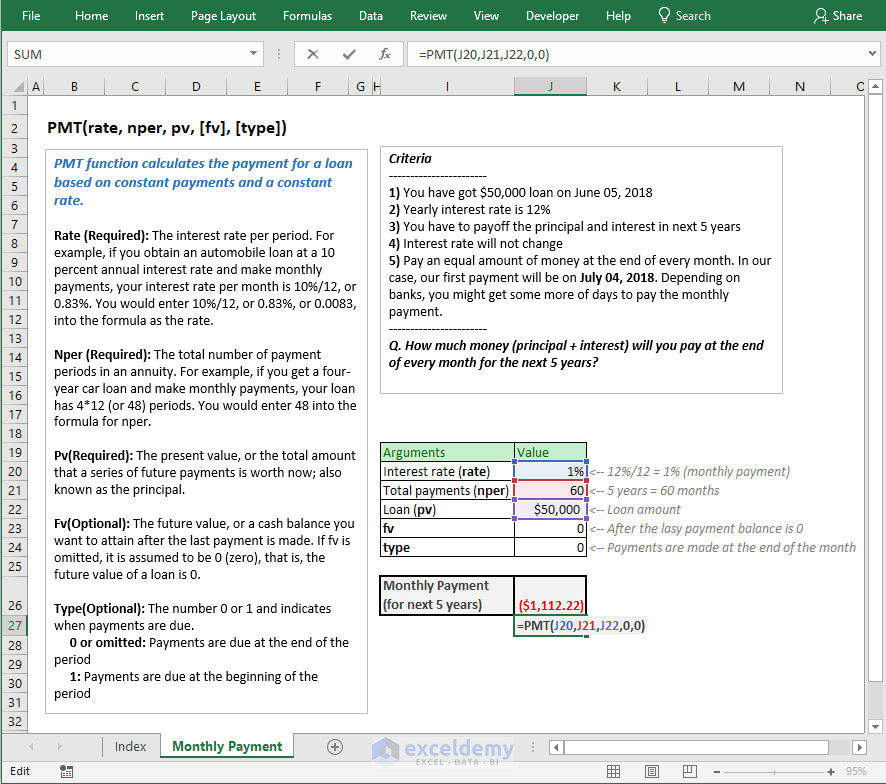Here are the criteria:

• You have got \$50,000 loan on June 05, 2018
• Yearly interest rate of the loan is 12%
• You will get 5 years’ time to pay off the loan
• The set interest rate 12% will not change over the next 5 years for your loan
• You have to pay an equal amount of money at the end of every month. In this case, your first payment will be on 4th July 2018 (end of the month). In reality, most of the banks will give you some more days to pay the monthly payment.

Here what we are going to find:

How much money (principal + interest) will you pay at the end of every month for the next 5 years?

You get the answer from the above image: (\$1112.22) every month.

By default, the amount is shown in the negative format as it is cash outflow. You can change it to positive by just adding a negative sign after the equal sign in the formula.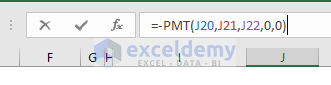And here is the result. The value is now positive.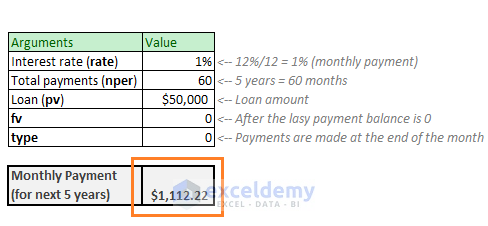Excel’s PMT function will output you the monthly payment for these types of cases.

So, let’s understand how PMT function works.

## Syntax of Excel PMT Function

Here goes the syntax of Excel’s PMT function:

PMT(rate, nper, pv, [fv], [type])

So, PMT function has 5 arguments. 3 of them (rate, nper, pv) are required, and 2 of them (fv, type) are optional.

 Argument Description rate (Required) Interest rate per period. Say, you got a loan at the yearly interest rate of 12%.Make payment monthly. Per period interest rate is 12%/12 = 1%.Make payment quarterly (every 3 months). Per period interest rate is 12%/4 = 3%Make payment half yearly (every 6 months). Per period interest rate is 12%/2 = 6% nper (Required) The total number of payment periods. Say, you got the above loan for next 5 years.Make payments monthly: Number of payments = 5*12 = 60Make payments quarterly: Number of payments = 5*4 = 20Make payments half yearly: Number of payments = 5*2 = 10 pv (Required) The present value. Simply, it is the loan amount you receive. fv (Optional) Future value. When you will calculate the loan payment, in most cases, this value will be 0. As at the end of your last payment, there will be no balance with the bank. If you don’t use this value, PMT will assume this value as 0. type (Optional) Type takes two values:0 or omitted: When you use 0 or omit this argument it means that payments are made (or due) at the end of the period.1: When the type is 1, it means payment will be due at the beginning of the loan period.

## Let’s check out the mathematics behind the scene

I know it is convenient to use the Excel’s PMT function to find out the monthly payments of a loan. But what if you want to know how the whole formula works behind the scene?

I will not show you the complex mathematics formula here like this one:

M = (P*i)/(q*(1-(1+(i/q))^(-n*q)))

Here,

• M is monthly payments
• P is the Principal amount
• i is the Interest rate
• q is the number of times a year you will make the payments
• n is the number of years you get to pay off the whole loan and its interest

I will not show you how this formula has got this face after passing several phases before.

What I am going to show is a clear understanding of the PMT function and its internal states. I found it enjoying when I did it. I hope you will also like it.

In the following image, you’re seeing some data for a loan you took from a bank:

• Yearly interest rate is 12%. So, per month interest rate is 12%/12 = 1%. So, PMT function’s rate argument is 1%.
• Principal amount, the amount you took from the bank is \$10,000. So, PMT function’s pv is 10,000.
• Number of years you’re getting to pay off the Principal and Interest is 3 years. This is a monthly payment, so the total number of periods you will get is 3 years x 12 = 36 months. So, nper is 60.

These are the 3 required arguments of PMT function.

And in cell C10, we have used the PMT function and got the value \$332.14. The value is positive as I have used negative sign (-ve) before the PMT function. Otherwise, PMT function gives negative values.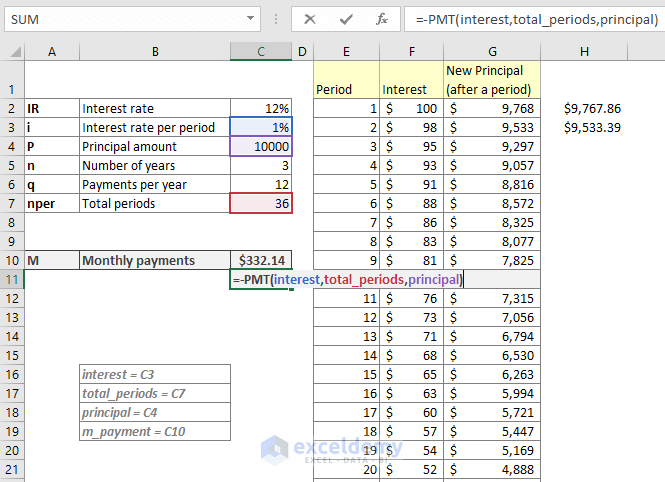Now the enjoying part. I will show you how the whole thing actually works behind the scene.

Think along with me. You will also enjoy this part.

### 1st payment done. What happens actually?

When you will make your first payment, you will pay the interest for 1 month (compounding interest) and some of the principal amount.

Our principal is \$10,000

One-month interest rate is 1% (bankers are clever, they divide the yearly rate by 12, so you have to pay higher than should pay).

So, one-month interest is \$10,000*1% = \$100

You’re paying \$332.14 at the end of first period or month.

So, you’re paying principal = \$332.14 – \$100 = \$232.14.

Then your new/residual principal will be = \$10,000 – \$232.14 = \$9767.86.

In the image, the value 9768 (rounded value of 9767.86) is showing in the cell G2 and the formula is showing on the right.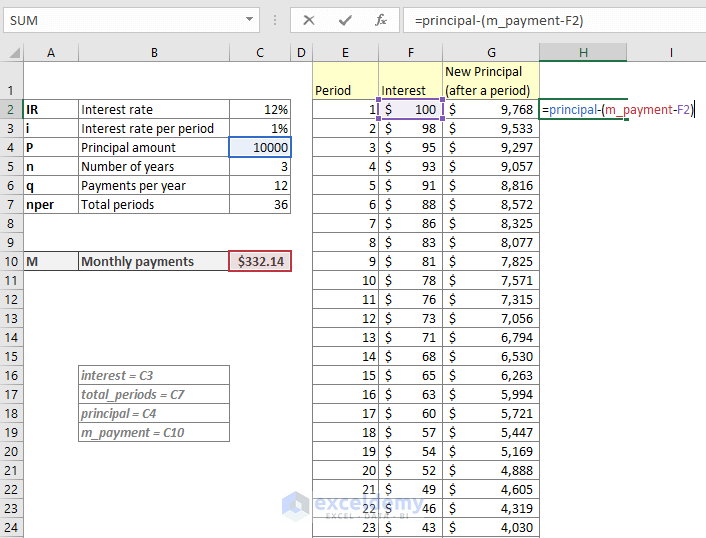### 2nd Payments done. What happens behind the scene?

When you’re making your second payment, you’re going to pay your interest based on the first-period-end principal amount and that is 9767.86.

So, you pay interest 9767.86*1% = 97.6786

Your monthly payment is same and it is 332.14.

So, you pay principal = 332.14 – 97.6786 = 234.46

Then at the end of the 2nd payment, your new or residual principal will be = 9767.86 – 234.46 = 9533.40

See the following image. Also, take a look at the formula that I have used to get this value.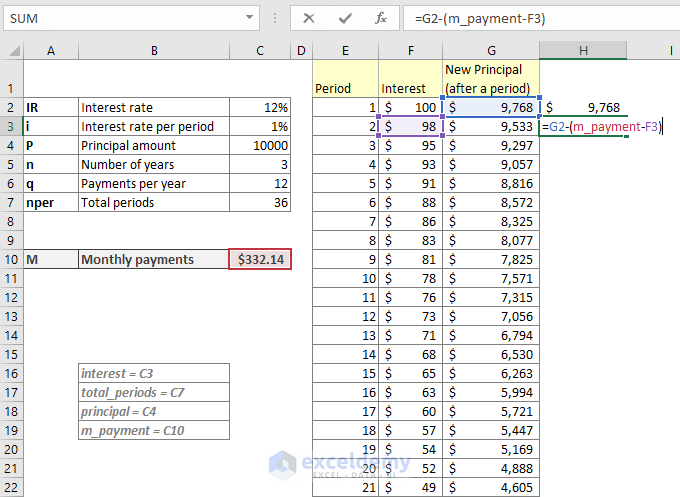We have used this formula in cell G3: =G2-(m_payment-F3)

m_payment = C10, it denotes the monthly payment

And this formula in cell F3 is to calculate the interest based on last principal: =G2*interest

Interest = C3, it denotes the monthly interest rate.

Now we copy down these two formulas for the rest of cells in the column and we get the following result.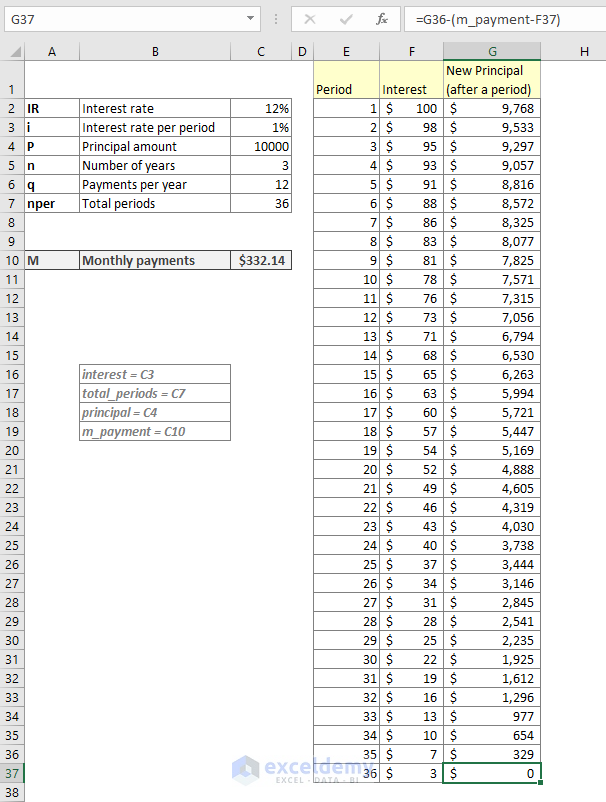Check all the iterations or the payment periods. You will find, in details, what is actually happening every period.

When you will pay your last payment (36th period), you will pay the interest of amount only \$3 and the previous period’s residual principal 329. So, your last payment is 329 + 3 = 332

## Calculating monthly payment by using the direct mathematical formula in Excel

This is the mathematical formula that calculates monthly payments:

M = (P*i)/(q*(1-(1+(i/q))^(-n*q)))

Here,

• M is monthly payments
• P is the Principal amount
• i is the Interest rate
• q is the number of times a year you will make the payments
• n is the number of years you get to pay off the whole loan and its interest

We can use this formula in Excel to find the monthly payments. Check out the following image.

In cell D7, we have used this formula: =(P*i)/(q*(1-(1+(i/q))^(-n*q))) and in cell D8, we have used this formula: =PMT(i/12,n*q,P,0,0). Both cells are giving the same results. PMT is giving negative value as it is cash outflow. But the numeric value is same.

Where, P = D2, i = D3, n = D4, q = D5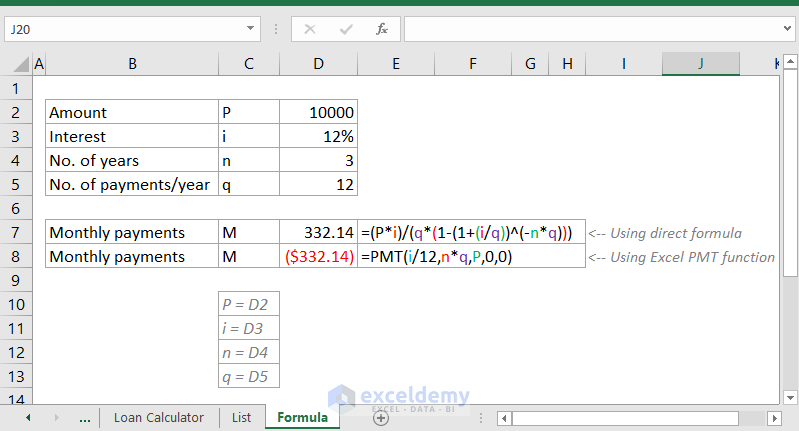## What if payment is done monthly but the interest rate is compounded semi-annually?

Let’s show you something different than so far, we have done.

Check out this scenario:

• Loan amount \$10,000
• Interest rate 12%
• Monthly payment
• But the interest rate is compounded semi-annually
• Payment periods 3 years = 36 months

A little bit critical case.

Think along with me:

• The interest rate will be compounded semi-annually (in every 6 months), right? So, divide 12% by 2 that returns 6%
• Now payments are monthly. So, over the 6 months of payments, you will pay overall 6% interest rate. If you think mathematically then, it will be like (1+x)^6 = 1.06 where is x is your monthly interest over the 6 months of payments. It is easy now to calculate the value of x from this equation => x = 1.06^(1/6) – 1 = 0.00975879. So, the value of x is 0.00975879

### Why cannot we divide the interest rate by 12

We cannot divide the interest rate by 12 when interest compounding is happening two times a year. If you do, you will pay more interest.

For example, say we divide the interest rate 12% by 12 (=1) and calculate the interest for 6 months.

It is: 10,000 (1+1/100)^6 – 10000 = 615.20. So, we are paying total \$615.20 interest when we are compounding the interest rate every month. At the end of 6 months, we are paying actually 6.15% interest

But in our case, we should pay 6% after 6 months.

Let’s use now the newly calculate interest rate (0.975879%) for this calculation.

10,000*(1+0.975879/100)^6 – 10000 = 600

So, with the newly calculated interest rate, we are paying overall 6% interest rate at the end of 6 months.

I hope this logic is now clear to you.

### Let’s calculate now the monthly payment for a semi-annually compounded interest rate

This was our data.

• Loan amount \$10,000
• Interest rate 12%
• Monthly payment
• But the interest rate is compounded semi-annually
• Payment periods 3 years = 36 months

And this is the solution.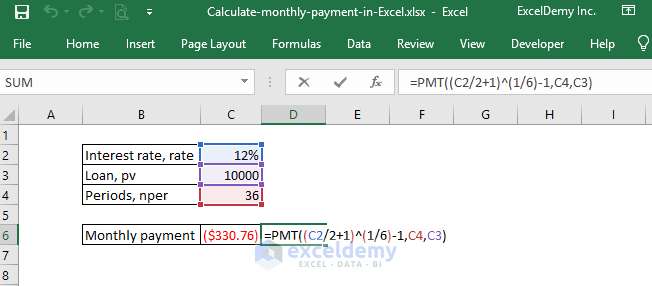We have used this formula in cell C6 = PMT((C2/2+1)^(1/6)-1,C4,C3)

Except for the rate argument, (C2/2+1)^(1/6)-1, you can easily understand the other arguments.

Let’s explain the rate argument:

• The value of C2 is 12%, so C2/2 = 12%/2 = 6%
• C2/2+1 = 6% + 1 = 1.06
• So, this part of the formula comes into this form: 06^(1/6) – 1 that results in the value 0.00975879.

So, this is how you can calculate the monthly payments of a loan when the interest rate is compounded semi-annually.

## Let’s make a complete loan calculator using Excel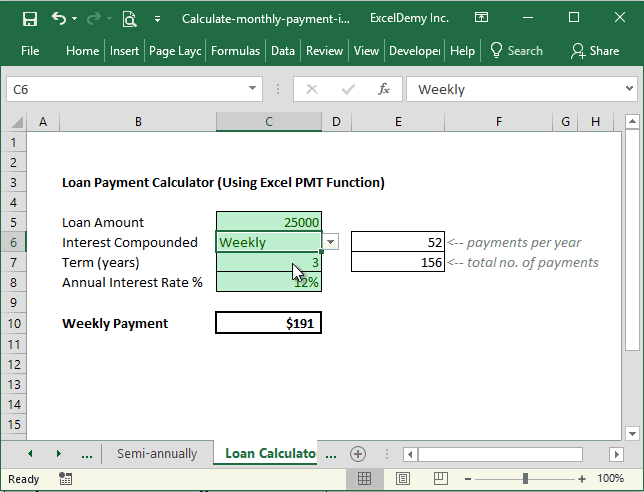Using the above Excel calculator, you can easily calculate any kind of loan payment, it can be weekly, monthly, Bi-weekly, etc.

We have used a VLOOKUP table like the following.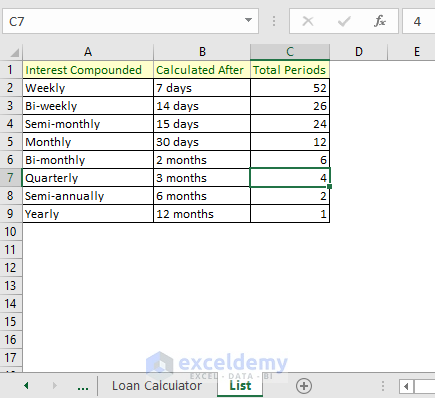Using this VLOOKUP table, we have created a formula in cell E6: = VLOOKUP(C6,List!A1:C9,3,0)

This formula finds out the number of payments per year.

Then we have calculated the total no. of payments in cell E7: E6*C7

So, these are my ways of calculating monthly payments for loan taken for several years. If you have any feedback, please let me know in the comment box.

## Related Online Courses

Hello!
Welcome to my Excel blog! It took me some time to be a fan of Excel. But now I am a die-hard fan of MS Excel. I learn new ways of doing things with Excel and share here. Not only how to guide on Excel, but you will get also topics on Finance, Statistics, Data Analysis, and BI. Stay tuned!

1. Replyrupy Apr 2, 2019 at 9:45 PM

i was thinking if you can make a dashboard for a few rental property just the thought

• ReplyJun 20, 2019 at 7:19 PM

Thanks for the suggestion 🙂 I will keep it on my list.

2. ReplyLawson lee Dec 1, 2019 at 8:48 AM

Do you have daily rest interest calculation? I don’t see in your worksheet.
Thanknyou

3. ReplyLawson lee Dec 1, 2019 at 8:51 AM

In case it wasn’t posted.
Home loan has daily compounding interest calculation.
Thank you

4. Replywasim Dec 13, 2019 at 10:28 PM

Would you mind if you show how to calculate interest compounded monthly on an outstanding late invoices.
for example, several invoices haven’t been paid after the due date, for a number of customers, and we’re going to charge 7% (Yearly), compounded monthly. how to keep track for the ongoing balance on a table for all customers ?
Hope you can find way to make it on one table..
Thank you …

• ReplyDec 14, 2019 at 7:28 PM

Hello Wasim,
I am keeping this on my to-do list. Will try to give you a solution of your problem.
Thanks.